# Mirror Images

The figure obtained by putting a mirror in front of the real object is known as mirror image or we can say that the reflection of an object into the mirror is called its mirror image.

Mirror Image is the image or the reflection of an object into a mirror when that object is placed near it.

Position of Mirror

There are two positions of mirror to get the image from it which are as follow

1. When Mirror is Placed Vertically

When a mirror is placed to the left or right of the object, then standard form of mirror image is formed. Here, the Left Hand Side (LHS) and Right Hand Side (RHS) of an object interchange their places while top and bottom remains at their position. Vertical mirror image is obtained by lateral inversion of the object.

The figure given below will help you to understand the formation of image formed by a vertical mirror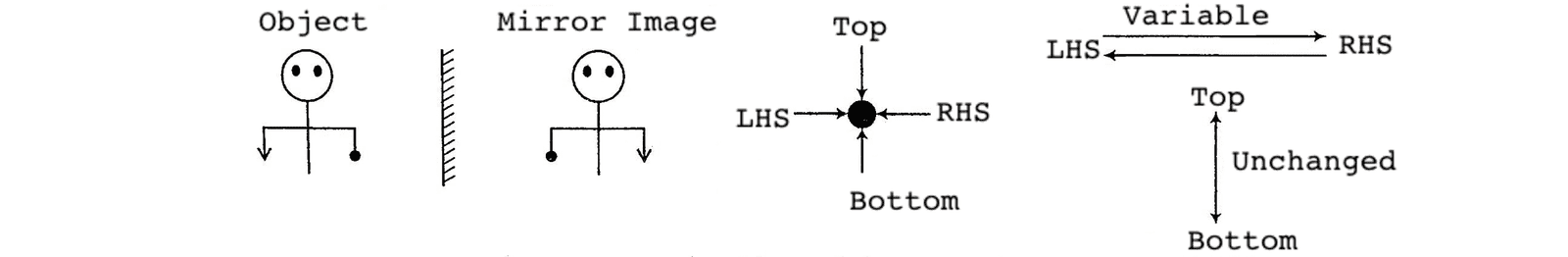In the above figure, you can see clearly that Left Hand Side (LHS) and Right Hand Side (RHS) have interchanged their position but top and bottom remains at the same place.

2. When Mirror is Placed Horizontally

When a mirror is placed at the top or bottom of the object, then the image , so obtained is like the water image. Here, the top and bottom of an object interchange their position.
Horizontal mirror image is obtained by vertical inversion of the object.

The figure given below will help you to understand the formation of image formed by a horizontal mirror.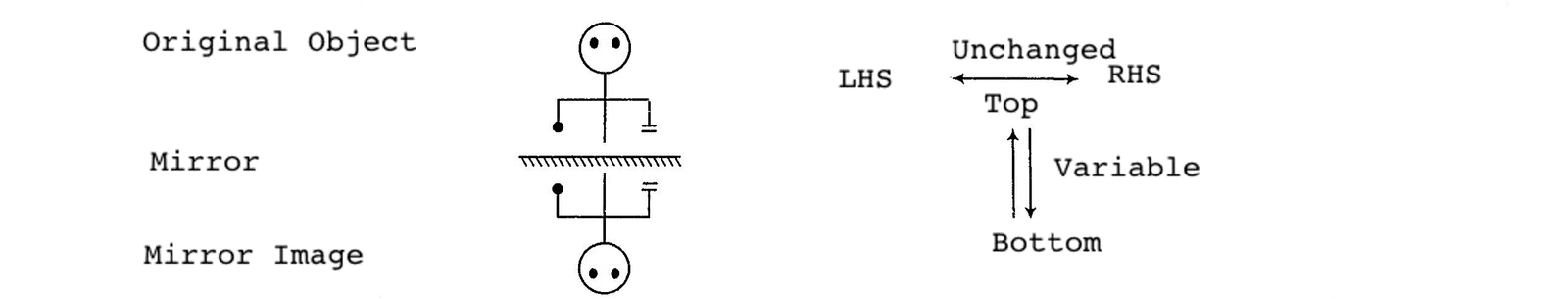In the above figure, you can see LHS and RHS of the image remains unchanged but the top and bottom interchange their positions.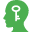Memory Add-ons

In mirror image, if the position of mirror is not given, then always consider mirror to be placed on right side.

Mirror Images of English Alphabet Letters and Numbers

Images of capital and small letters of English Alphabet and numbers formed by vertical mirror are given below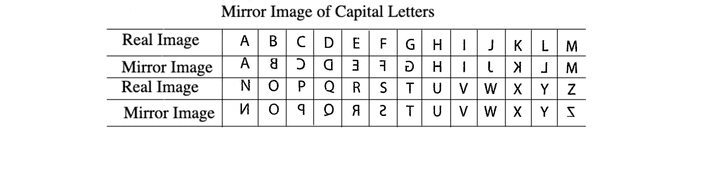Note : The letters ---- A, H, I, M, O, T, U, V, W, X and Y have the same mirror images i.e. their left hand and right hand sides do not appear to change their positions.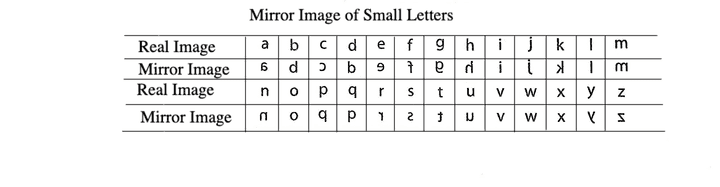Note : The letters --- i, l, o, v, w and x have the same mirror images i.e. their left hand and right hand sides do not appear to change their positions.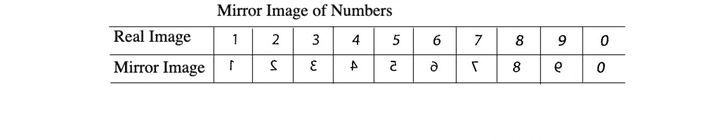Note : Numbers 0 and 8 have the same mirror images.

Different types of questions covered in this section are as follows

1. Based on Vertical Mirror
2. Based on Horizontal Mirror
3. Mirror image of Letters and Numbers
4. Mirror Image of Clocks

In case of standard form of mirror image i.e., when the mirror is placed vertically, the object gets laterally inverted. In order words, the Left Hand Side (LHS) and Right Hand Side (RHS) of an object interchange their places while top and button remain the same.

Let us see an example to get a better idea about the concept of mirror images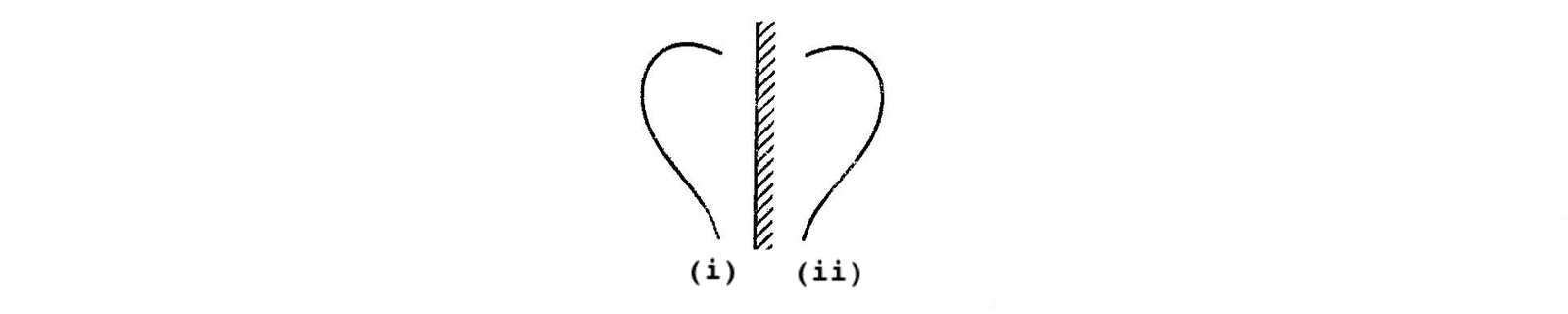Here, Fig. (ii) is the mirror image of Fig. (i). On combining these two figures.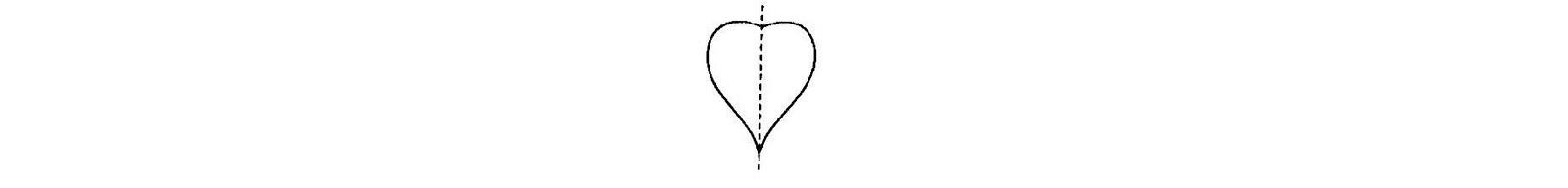We get a 'heart' shaped figure which is symmetrical along an imaginary line which is used in place of the mirror

Objects having Different Mirror Images

Mostly the mirror image of a figure is different from the original figure which is because of the dissimilarity in the left and right halves of the figure. This can be better understood with the help of the below examples.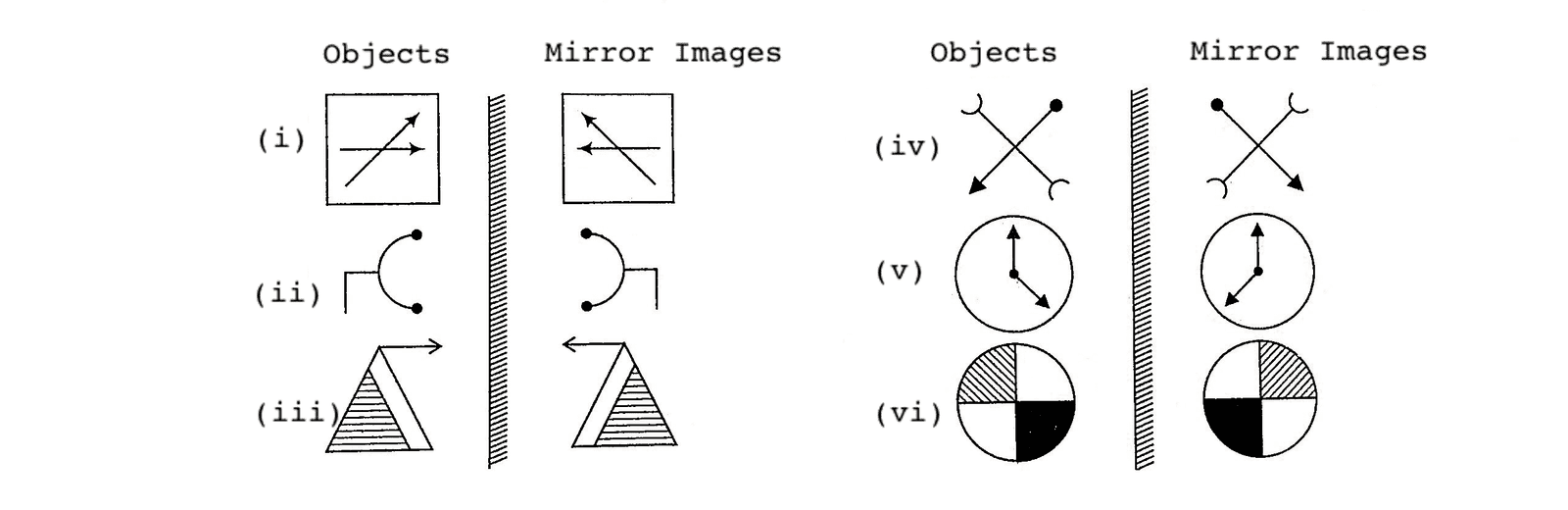Objects having Identical Mirror Images

Sometimes it happens that mirror image formed is identical to the original figure. This is the case when LHS and RHS halves of the figure are similar to each other but in opposite directions. This can be better understood with the help of the below examples.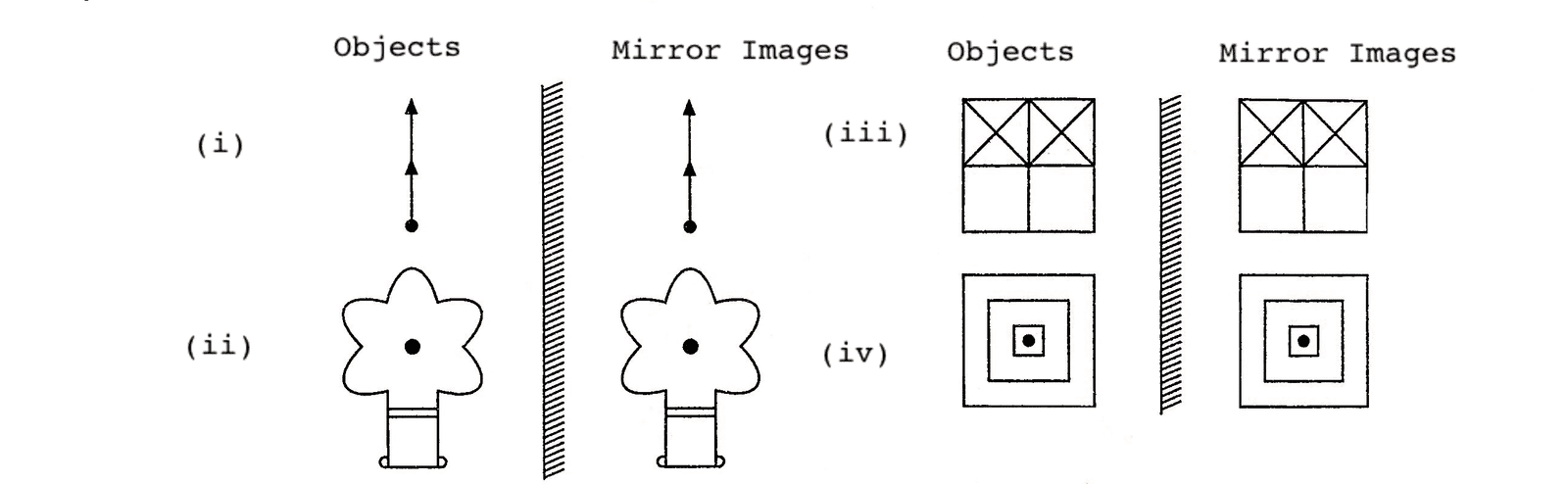In the above discussion, the concept has been given about standard form of mirror image in which vertical mirror is used.

Now, we will discuss different types of questions based on placing of mirror on which questions are asked in various exams

## Types of questions

There are four types of questions, which are generally asked in various competitive exams, regarding mirror images.

Type #1: Based on Vertical Mirror

In such questions a vertical mirror is placed to the left or right of the object and standard form of mirror image is formed where the Left Hand Side (LHS) and Right Hand Side (RHS) of the object interchange their places while top and bottom remain unchanged.To get standard form of mirror image, the mirror is placed vertically to the left or right of the object and in both the cases, the image obtained is same.

case I  When the mirror is placed vertically to the right of the object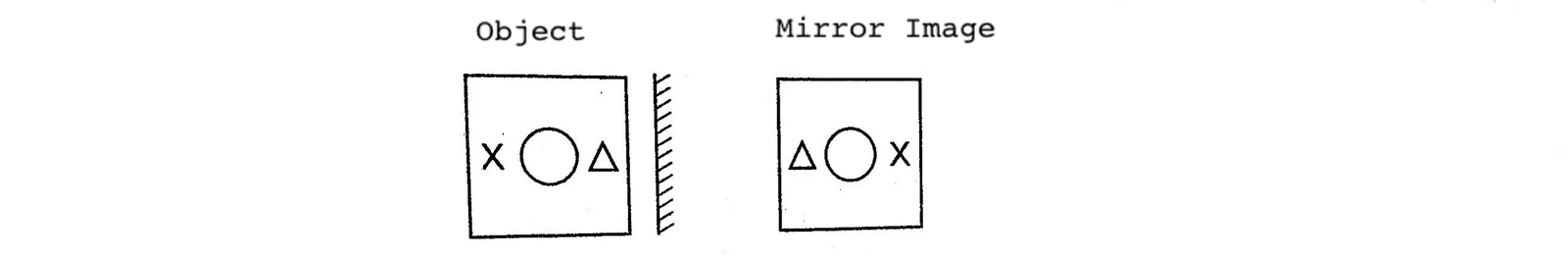case II  When the mirror is placed vertically to the left of the object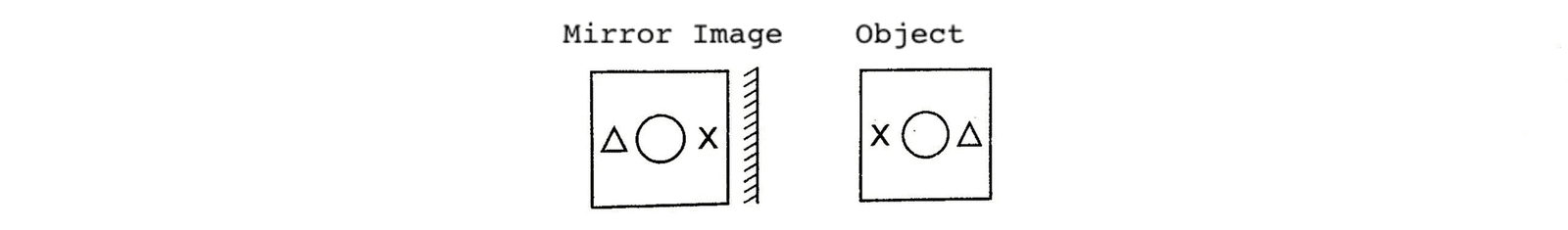Memory Add-ons

For finding mirror image of object/figure, try to overlap the figure given in the options, along the mirror as the axis, then the option figure which exactly overlaps the original figure is the correct answer.

Your will get a better idea from the following examples about the various types of questions asked

Directions (Example Nos. 1-2) In each of the following questions you are given a figure followed by four alternatives (a), (b), (c) , (d) and (e). Choose the alternatives which is closely resembles the mirror image of the given combination.

Example 1: Choose the alternatives which is closely resembles the mirror image of the given combination.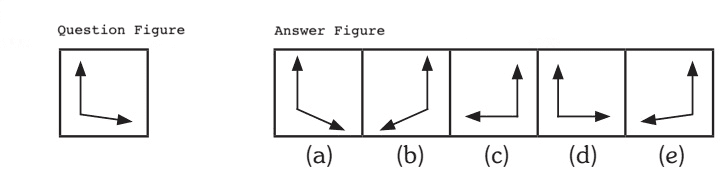Solution: (e) Here, the mirror is placed vertically on the RHS on the question figure. Hence, only the figure given in answer figure (e) would be obtained as the correct mirror image.

Example 2: Choose the alternatives which is closely resembles the mirror image of the given combination.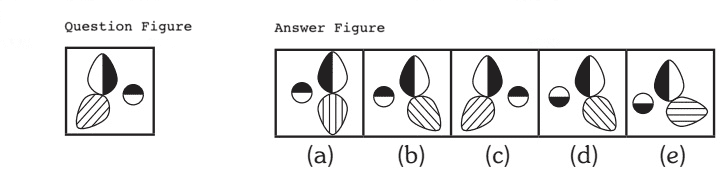Solution: (b) Here, the mirror is placed vertically on the RHS on the question figure. Hence, only the figure given in answer figure (b) would be obtained as the correct mirror image.

Type #2: Based on Horizontal Mirror

In such questions a horizontal mirror is placed at the top or bottom of the object and the image, so obtained is like the water image. In this type of question, LHS and RHS remain unchanged.

Let us see the two cases given below

case I When the mirror is placed horizontally at the top of the object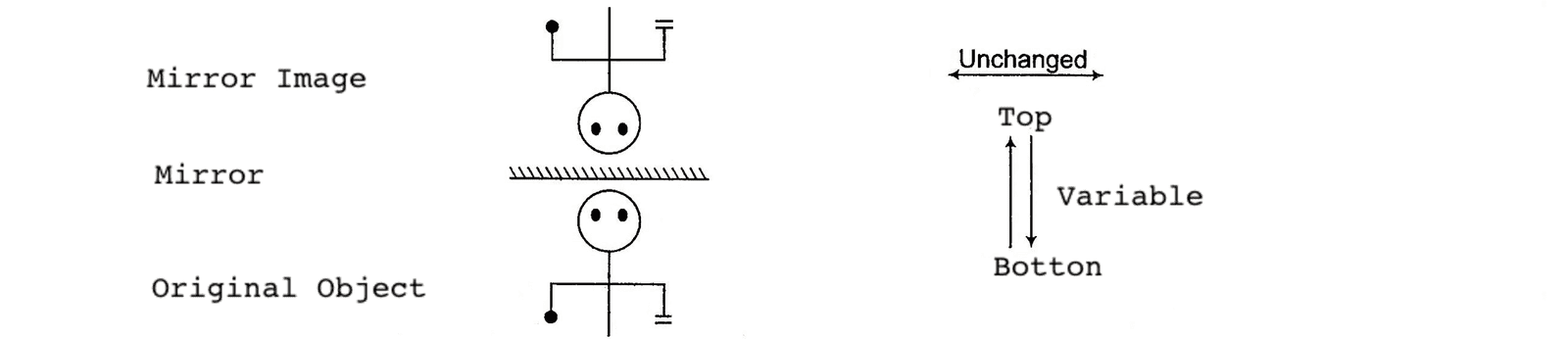case II When the mirror is placed horizontally at the bottom of the objectThe following examples will give you a better idea about such type of questions

Directions (Example Nos. 3-5) In each of the following questions, choose the correct mirror image from the alternative (a), (b), (c), and (d) when mirror is placed along the line AB.

Example 3: Choose the correct mirror image from the answer figures (a), (b), (c) and (d).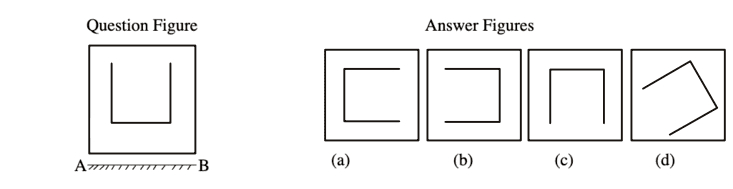Solution: (c) Here, the mirror is placed horizontally at AB i.e., at the bottom of the question figure. Hence, only answer figure (c) looks like the water image of the question figure. It means, option (c) is the vertically inverted form of question figure in which top and bottom interchange places while LHS and RHS remain the same.

Example 4: Choose the correct mirror image from the answer figures (a), (b), (c) and (d).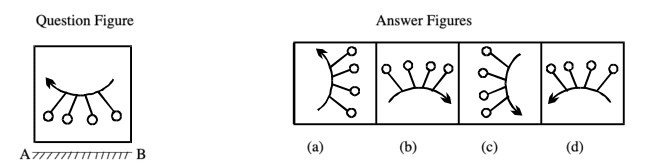Solution: (d) Here, the mirror is placed horizontally at AB i.e., at the bottom of the question figure. Hence, only answer figure (c) looks like the water image of the question figure. It means, option (c) is the vertically inverted form of question figure in which top and bottom interchange places while LHS and RHS remain the same.

Example 5: Choose the correct mirror image from the answer figures (a), (b), (c) and (d).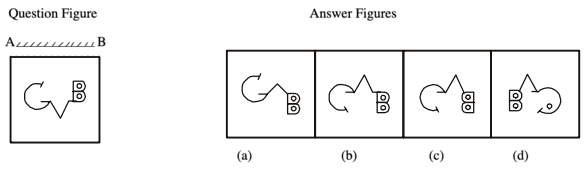Solution: (b) Here, the mirror is placed horizontally at AB on the top of the question figure.Hence only answer figure (b) is the vertically inverted form of question figure in which top and bottom interchange places while LHS and RHS remain the same.

Type #3: Mirror Image of Letters and Numbers

In this type of questions, a number, letter or a combination of letters and/or numbers is given and the candidates are required to find their mirror images out of given alternatives.

Directions (Example Nos. 6-9) In each of the following questions, you are given combination of letter's and/or numbers followed by four alternatives (a), (b), (c), and (d). Choose the alternative which most closely resembles the mirror image of the given combination.

Example 6: Choose the alternative which most closely resembles the mirror image of the given combination.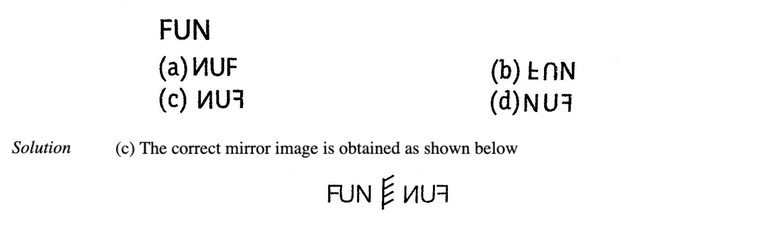Example 7: Choose the alternative which most closely resembles the mirror image of the given combination.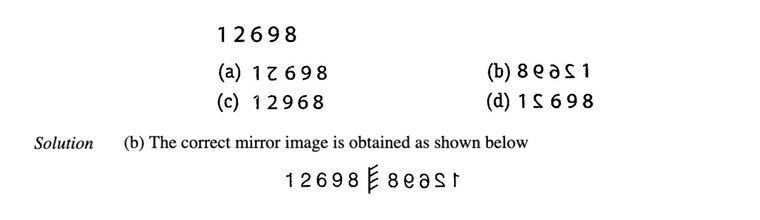Example 8: Choose the alternative which most closely resembles the mirror image of the given combination.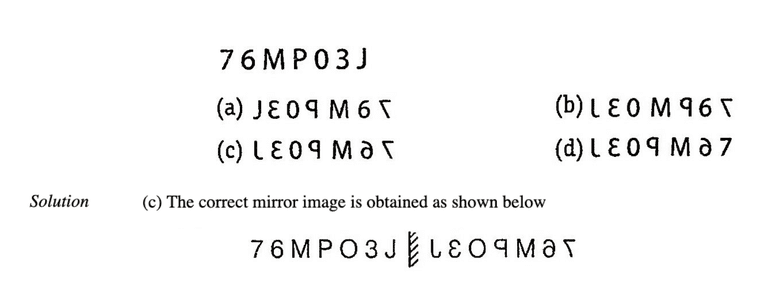Example 9: Choose the alternative which most closely resembles the mirror image of the given combination.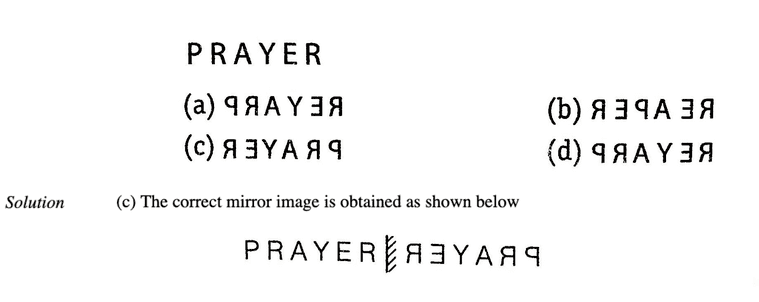Type #4: Mirror Image of Clocks

In this type of questions, a candidate has to find the original positions of the hour hand and minute hand of a clock from the positions of the hour hand and the minute hand as seen in a mirror. On the basis of time indicated by mirror image of the clock, we have to find the actual time in the clock. The following examples will help understand the concept better.

Example 10: A clock seen through a mirror show quarter to three. What is the correct time shown by the clock?

(a) 8 : 15    (b) 9 : 12    (c) 8 : 17    (d) 9 : 15

Solution: (d) Here,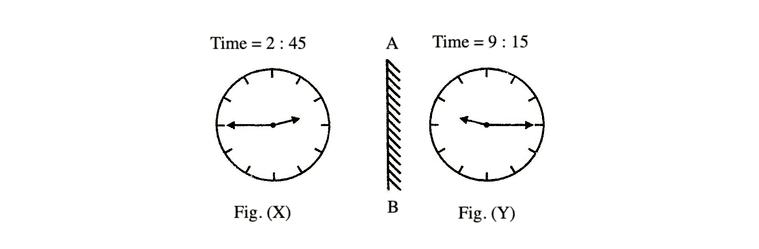It is clear from Fix. (X) that if seen through a mirror the time indicated by clock is 2 .45. When the mirror is placed vertically at AB, the mirror image i.e., Fig. (Y) shows the actual time in the clock i.e., (9 : 15).

Example 11: A clock seen through a mirror show quarter past three. What is the correct time shown by the clock?

(a) 9 : 45    (b) 9 : 15    (c) 8 : 45    (d) 3 : 15

Solution: (c) Here,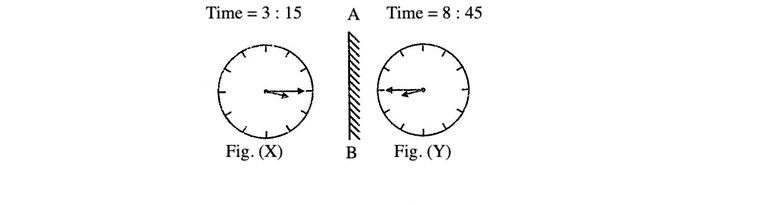It is clear from Fix. (X) that if seen through a mirror the time indicated by clock is 3 .15. When the mirror is placed vertically at AB, the mirror image i.e., Fig. (Y) shows the actual time in the clock i.e., (8 : 45).Memory Add-ons

In case of words, letters and clocks, the mirror is always kept in standard form i.e., vertically to obtain the mirror image unless otherwise stated.

LearnFrenzy provides you lots of fully solved Mirror Images Non Verbal Reasoning Questions and Answers with explanation.